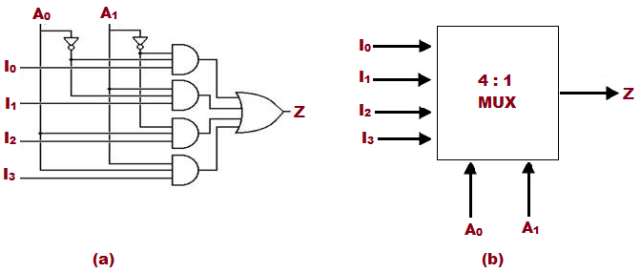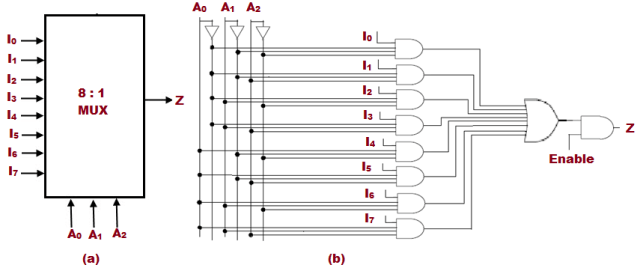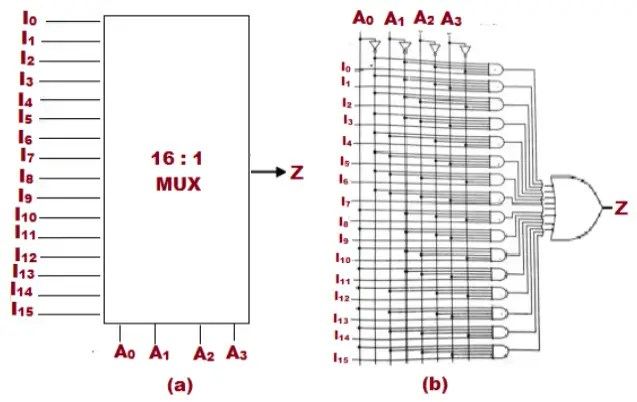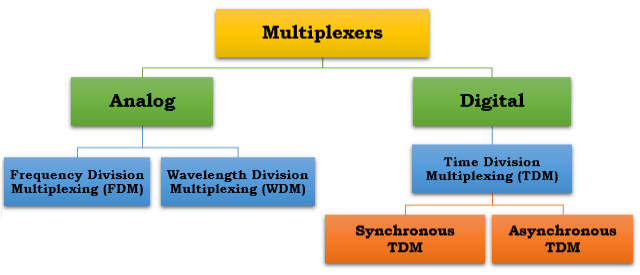Tuesday, December 7, 2021
HomeDigital TechnologyMultiplexer (Mux) - Types, Cascading, Multiplexing Techniques, Application

# Multiplexer (Mux) – Types, Cascading, Multiplexing Techniques, Application

Multiplexer is a combinational circuit that is used to switch either analog, digital or video signals. It is a simple circuit which accepts multiple analog signals or digital data streams and combines into one signal and transmits over a shared medium. This post will discuss about what is Multiplexer, its types, cascading of Multiplexers, Multiplexing techniques, applications, advantages and disadvantages.

## What is Multiplexer

Multiplexer is a combinational circuit which accepts multiple analog signals or digital data streams and combines into one signal and transmits over a shared medium.Fig. 1 – Schematic Diagram of 2:1 Mux

Let us understand its function in a simpler way. We have taken a 2:1 Mux as shown in Figure 1 above, where: –

• I0 and I1 are the two data inputs
• A is the control input
• Z is the single output

Possible Outcomes:

• If A = 0, then data input I0 will be connected to the output Z. Hence Z = I0
• If A = 1, then data input I1 will be connected to the output Z. Hence Z = I1

Based on control inputs, logic equation for the 2:1 MUX is derived i.e. Z = A’ I0 + A I1

## Types of Multiplexer

There are several types of Multiplexers and few are listed as follows:

• 2:1 Multiplexer
• 4:1 Multiplexer
• 8:1 Multiplexer
• 16:1 Multiplexer

### 2:1 Mux

The 2:1 Multiplexer consists of 2 data inputs, 1 control bit and 1 output bit. Iand I1 are the two input bits, A is the control bit or the select bit and output Z.Fig. 2 – (a) Block Diagram of 2:1 Mux (b) Logic Gate Diagram of 2:1 Mux

The logic equation for the 2:1 Multiplexer is Z = A’ I0 + AI1. Figure 2 above illustrates the pin diagram and circuit diagram of 2:1 Multiplexer.

### 4:1 Mux

The 4:1 Multiplexer consists of 4 data input bits, 2 control bits and 1 output bit. I0, I1, I2, I3 are the four input bits, A0 and A1 are the control bits and output is Z.Fig. 3 – (a) Block Diagram of 4:1 Mux (b) Logic Gate Diagram of 4:1 Mux

The logic equation of 4:1 Mux is Z = A’0A’1I0 + A’0A1I1 + A0A’1I2 + A0 A1 I3. Figure 3 above illustrates the pin diagram and circuit diagram of 4:1 Multiplexer.

### 8:1 Mux

The 8:1 Multiplexer consists of 8 data input bits, 3 control bits and 1 output bit. I0, I1, I2, I3, I­4, I5, I6, I7, I8 are the eight input bits and A0, A1 and A2 are the control bits and output is Z.Fig. 4 – (a) Block Diagram of 8:1 Mux (b) Logic Gate Diagram of 8:1 Mux

The logic equation of 8:1 multiplexer is Z= A’0 A’1 A2’ I0 + A’0A1A2 I1+ ……(so on). Figure 4 above illustrates the pin diagram and circuit diagram of 8:1 Mux.

### 16:1 Mux

The 16:1 Multiplexer consists of 16 data input bits, 4 control bits and 1 output bit. I0, I1, I2, I3, I­4, I5, I6, I7, I8 are the sixteen input bits, A0, A1, A2 and A3 are the control bits and output is Z.Fig. 5 – (a) Block Diagram of 16:1 Mux (b) Logic Gate Diagram of 16:1 Mux

The logic equation of 16:1 multiplexer is Z= Z = A’0A’1 A2’ A3’ I0 + A’0A1A2A3I1+ …… (and so on). Figure 5 above illustrates the pin diagram and circuit diagram of 16:1 Mux.

Cascading refers to a process where large Multiplexers can be designed and implemented using smaller Multiplexers. Example: 8:1 Mux can be designed using two 4:1 Multiplexers and similarly it can be designed using four 2:1 Multiplexers as shown in the below figure.Fig. 6 – (a) 8:1 Mux Designed Using Two 4:1 Mux (b) 8:1 Mux Designed Using Four 2:1 Mux

## Classification of Multiplexing Techniques

Multiplexers are used in both Analog and Digital Multiplexing techniques. Multiplexers that are used for Analog applications are designed using relays and transistor switches. Multiplexers that are used for Digital applications are designed using standard Logic Gates.

### Analog Multiplexing Techniques

• Frequency Division Multiplexing
• Wavelength Division Multiplexing

### Digital Multiplexing Techniques

Digital Multiplexing is achieved by Time Division Multiplexing (TDM). Time Division Multiplexing (TDM) can be further classified into two types. They are:

• Synchronous TDM
• Asynchronous TDMFig. 7 – Classification of Multiplexing Techniques

## Applications of Multiplexer

The applications include:

• Multiplexers are used in Communication Systems like telephone networks, Satellite systems, Telemetry.
• Broadcasting of Radio and Television signals would have been impossible without multiplexers.
• Multiplexer is also used in data routing within the computer. For example: The address information found in a protocol header is used by the multiplexer to determine which data should be routed.
• Multiplexers are widely used in computer memory to fetch data from specified memory locations.
• Multiplexer is used as a switch setting Comparator and Function Generator.Fig. 8 – Application of Multiplexer

• Bandwidth is used effectively.
• A single line is used to transmit multiple signals.

In Analog or Digital transmission using multiplexers, synchronization of the transport signals becomes critical.

```Also Read:
Karnaugh Map (K-Map) – Minterm, Maxterm, Simplification & Applications
Logic Gates – Types, Working Principle, Application, Advantage
```Laxmi Ashrit
Laxmi is a B.E (Electronics & Communication) and has work experience in RelQ Software as Test Engineer and HP as Technical support executive. She is an author, editor and partner at Electricalfundablog.
RELATED ARTICLES